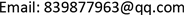1. 引言

2. 定子损耗计算

Parameters of machin

P F e = K h f B n α + K e f B n 2 (1)

P F e = K h ∑ v = 1 ∞ v f ( B v max α + B v min α ) + K e ∑ v = 1 ∞ ( v f ) 2 ( B v max 2 + B v min 2 ) (2)

3. 温度场计算

T S 1 = T S − λ n ∂ n | S 2 = q 0 − λ n ∂ n | S 1 = h ( T − T c ) } (4)

∂ ∂ x ( λ x x ∂ T ∂ x ) + ∂ ∂ y ( λ y y ∂ T ∂ y ) = q (5)

1热源不随温度变化；

2材料特性不随温度改变；

3槽内绕组、槽内气隙与绝缘纸、定子轴向导热系数分别进行等效计算；

4铁心端面、槽绝缘端面及铁心外表面的散热系数取经验值。

3.1. 生热率的求取

q s = P l o s s / S (6)

Heat generation rat

3.2. 定子的散热与导热

λ x = λ y = k F e λ 1 + ( 1 − k F e ) λ 0 (7)

λ R = ( δ c u + δ j ) λ c u λ j δ c u λ j + δ j λ c u (8)

α j = 1 + ω r 60000 × 10 6 (9)

α c = 28 ( 1 + v δ 0.5 ) v δ = 1 2 ω r } (10)

3.3. 水路结构的散热特性

α w = N u λ w d h (11)

d h = 4 A U (12)

N u = 0.12 ( R e 0.87 − 280 ) P r 0.4 × [ 1 + ( d / L ) 2 / 3 ] × ( P r / P w ) 0.11 (13)

R e = ρ W v w d h / u P r = u c P λ W } (14)

System resulting data of standard experimen

389

0.604

10号钢45

4. 温度场结果与分析

5. 结论

1) 由于高平硅钢片的铁损是非晶合金的4倍多，硅钢片定子的各个位置的温度都高于非晶合金。

2) 外加水冷散热效果明显。

3) 两种材料的最高温度都发生在齿顶部位，因此在实验时应更加关注定子齿部的温度变化；综上所述，在对定子温度进行检测时应关注定子齿根的温度变化。由于本文未搭建试验平台进行实验，无法计算结果的准确度。